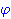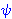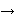New Foundations Explorer < Previous   Next > Nearby theorems Mirrors  >  Home  >  NFE Home  >  Th. List  >  simpli Unicode version

Theorem simpli 444
 Description: Inference eliminating a conjunct. (Contributed by NM, 15-Jun-1994.)
Hypothesis
Ref Expression
simpli.1Assertion
Ref Expression
simpliProof of Theorem simpli
StepHypRef Expression
1 simpli.1 . 22 simpl 443 . 231, 2ax-mp 8 1Colors of variables: wff setvar class Syntax hints:wa 358 This theorem was proved from axioms:  ax-1 5  ax-2 6  ax-3 7  ax-mp 8 This theorem depends on definitions:  df-bi 177  df-an 360 This theorem is referenced by: (None)
 Copyright terms: Public domain W3C validator